# Reading Hours on a Clock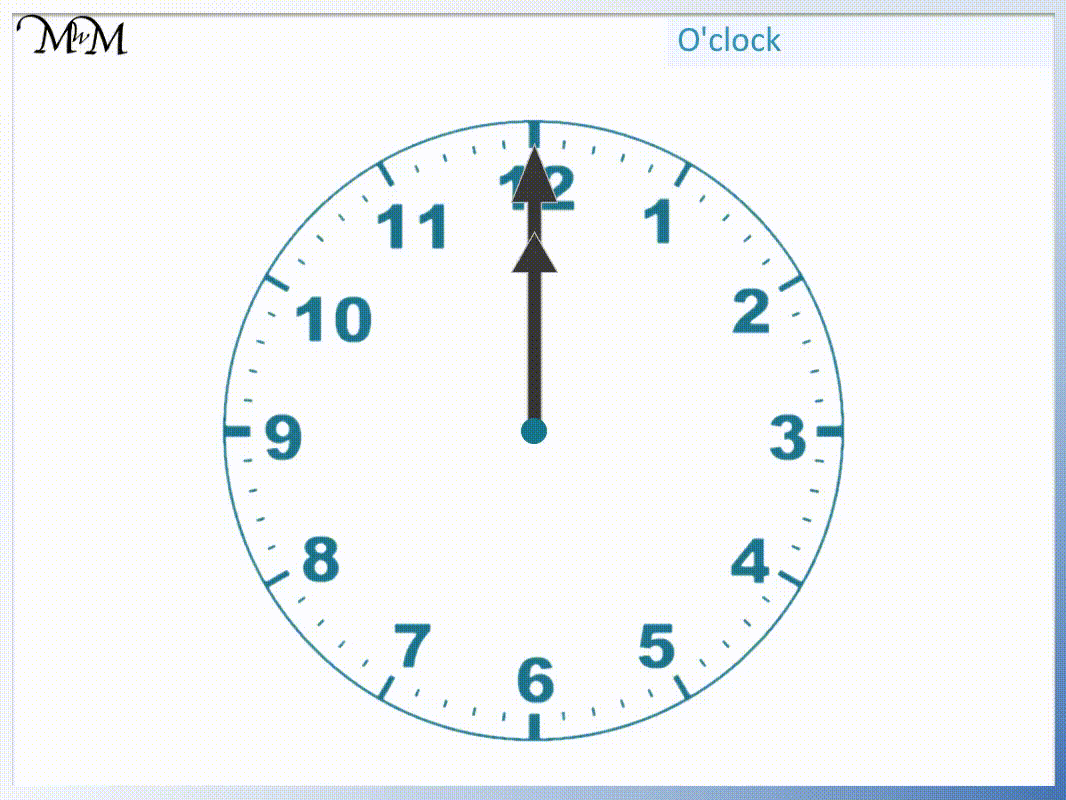• When the longer minute hand points upwards to the 12, then we have an hour.
• The numbers on the outside of the clock face show the different hours.
• The number that the shorter hour hand points at is what hour it is.
• We say o’clock after each number to say the time.
• When the hour hand points at the 1 we say that the time is 1 o’clock.
• When the hour hand points at 2 we say that the time is 2 o’clock etc.
• o’clock is a short way to say ‘of the clock’.
• So 3 o’clock is just a quick way to say the 3rd hour of the clock.
• Every hour the longer minute hand completes a full turn back to point at the 12.
• Every hour, the shorter hour hand moves from one number on the clock to the next.

The time is an exact hour if the longer minute hand points at the 12.

The shorter hour hand points at the number on the clock face which hour it is.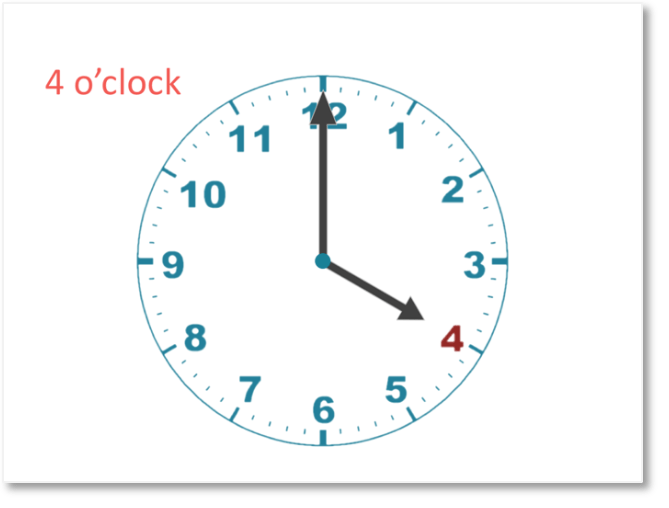• The longer minute hand is pointing to the 12 at the top of the clock face.
• This means that the time is an exact hour.
• The number on the clock face that the shorter hour hand points to is the hour.
• The hour hand points at 4 so it is 4 o’clock.# Telling the Time in Hours using a Clock

## Important Parts of a Clock Face

When first teaching someone to tell the time it is important that they first understand the different parts of a clock face.

A clock face is made up of 12 hours.

We start at 1 and count upwards until we get to twelve.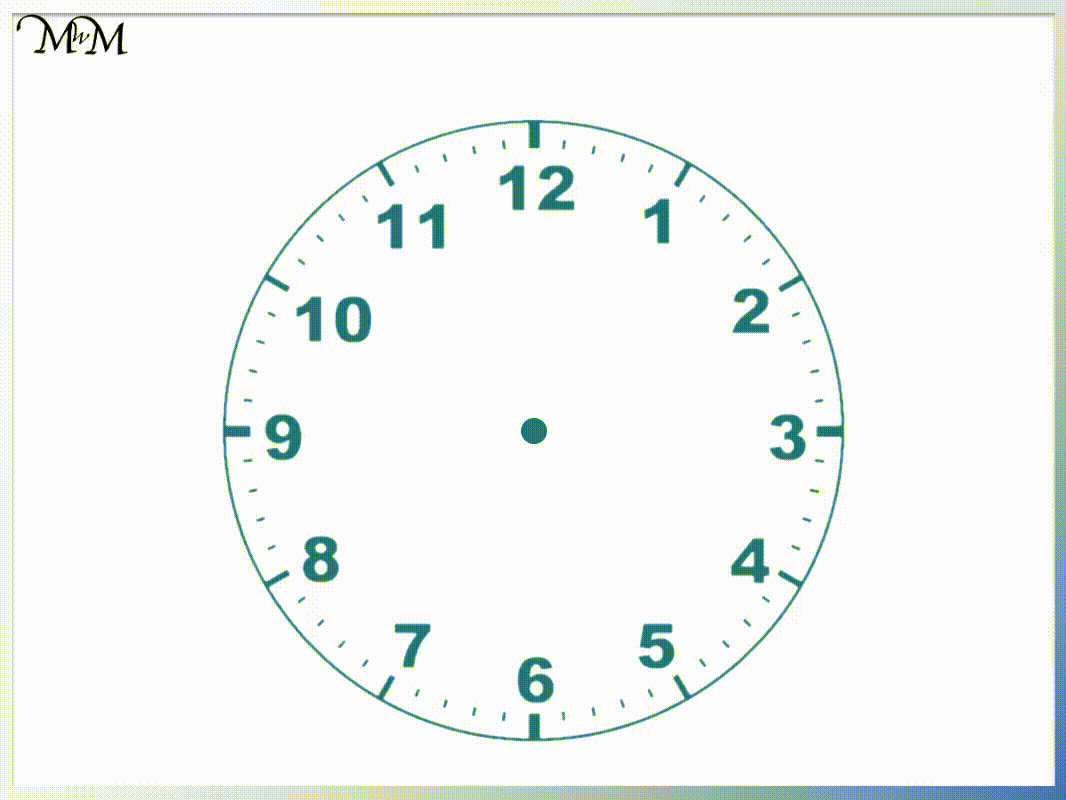When teaching time, you should count the numbers around the clock from 1 to 12 and you could get your child to point at each number as you count it.

Show them that after 12, we go back to 1 again. In each day we count from 1 to 12 twice. After we count to 12 the first time, we start again at 1 and count to 12 again.

A clock has 2 important hands that we use to read the time. One hand is longer than the other.

The longest hand is called the minute hand.

The shortest hand is called the hour hand.

Many watches and clocks actually have 3 hands in total. The third hand is the seconds hand but when first teaching time we can forget about this hand. This is because we usually read time to the nearest minute not the nearest second.The minute hand completes one full turn every hour.

The hour hand moves from one number to the next every hour.

24 hours make up one whole day. Because there are only 12 numbers on a clock face, the hour hand moves around the whole clock twice in one day. There are 12 hours in the morning and 12 hours in the evening.

The hands on the clock move around the clock face like so: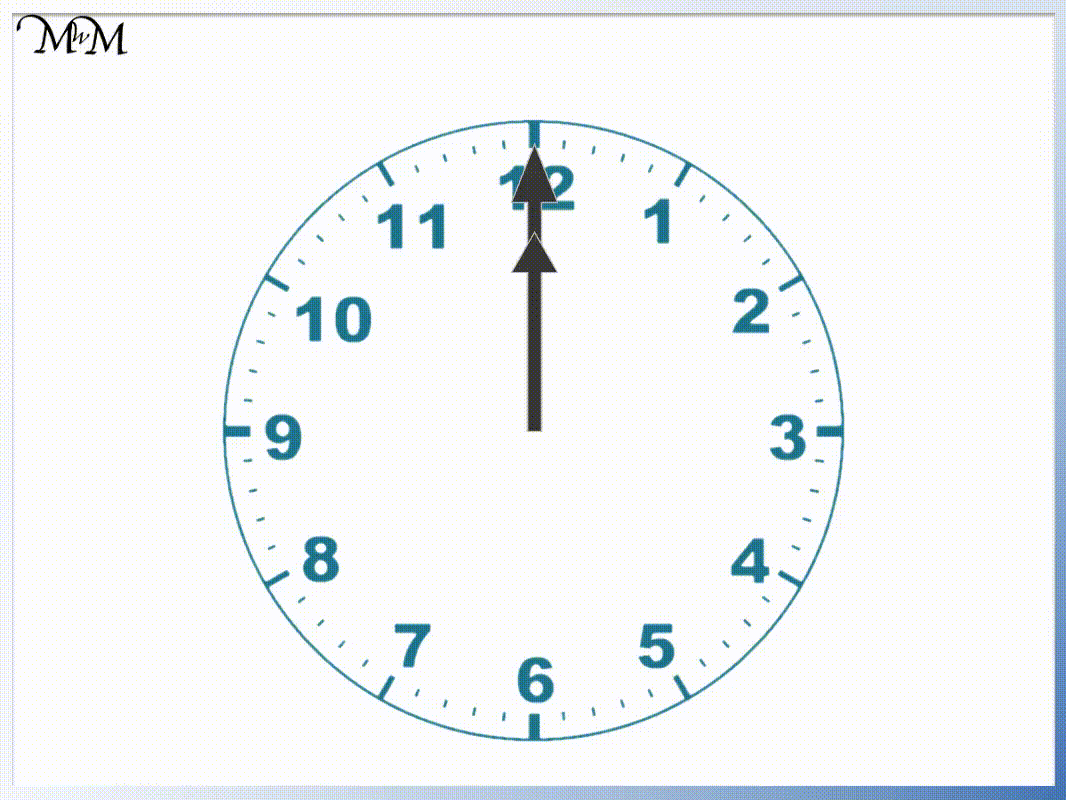The hands always rotate around in the same direction. From pointing at 12, the hands move around to the 1, then the 2, then the 3. We can remember the direction that the hands move by following the numbers on the clock face. The numbers go in order when the hands move in the correct direction.

The direction that the clock hands move is called clockwise. The opposite direction is called anticlockwise or counterclockwise. All of the hands on a clock always travel in a clockwise direction.## Reading Hours on a Clock

If the longer minute hand is pointing to the 12 then the time is an exact hour. The number that the shorter hour hand is pointing to tells us which hour it is.

We read this number and say o’clock after it to show that the time is an exact hour.

A new day begins with both hands on the clock pointing to 12. They both point directly upwards.

In one hour, the longer minute hand completes a full turn of the clock face and the shorter hour hand moves from one number on the clock to the next.

Here are the hours in a day shown on a clock face.Starting with the hands pointing at 12, the first number we come to is 1.

The minute hand has completed one full turn and is pointing back at the 12.

The hour hand has moved from 12 to 1.In this example, the longer minute hand is pointing to the 12 so we know that it is an exact hour. The shorter hour hand is pointing at the 1 so we know that the hour is 1. We put the word o’clock after the number when the time is an exact hour.

We read this time as 1 o’clock.

After the minute hand completes one more full turn of the clock, the next hour we get to is 2 o’clock.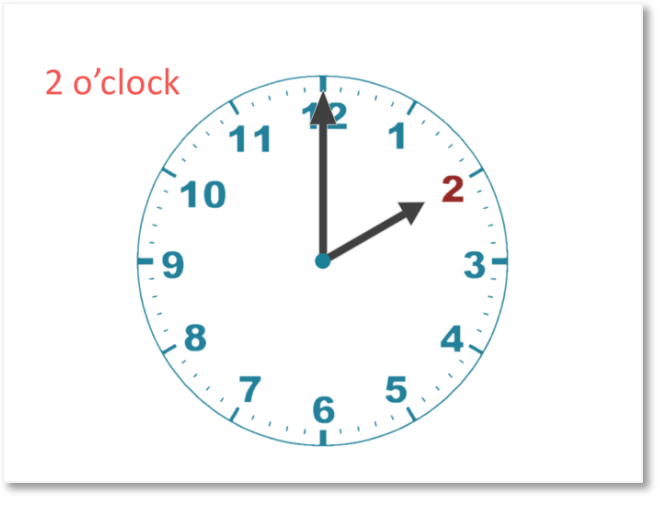We can see that the minute hand is pointing directly upwards at the 12 so we know that it is an exact hour.

The hour is 2 o’clock because the shorter hour hand points at the 2.

The next hour is 3 o’clock.The next hour is 4 o’clock.Then we have 5 o’clock, 6 o’clock, 7 o’clock, 8 o’clock, 9 o’clock, 10 o’clock, 11 o’clock and finally 12 o’clock.At twelve o’clock both hands are pointing directly upwards at the 12.

The longer minute hand must point at the twelve because it is an exact hour.

The hour hand points at the 12 to show that it is 12 o’clock.

After 12 o’clock, we have 1 o’clock again and the hour cycle repeats itself.

This cycle happens twice in a complete day. Once in the morning and once in the evening.

When teaching time and your child is learning the hours on the clock, you should show them the clock at each new hour and ask them what time it is. This familiarises them with using the hour hand and how long an hour lasts.Now try our lesson on Half Past the Hour where we learn how to read half past the hour on a clock.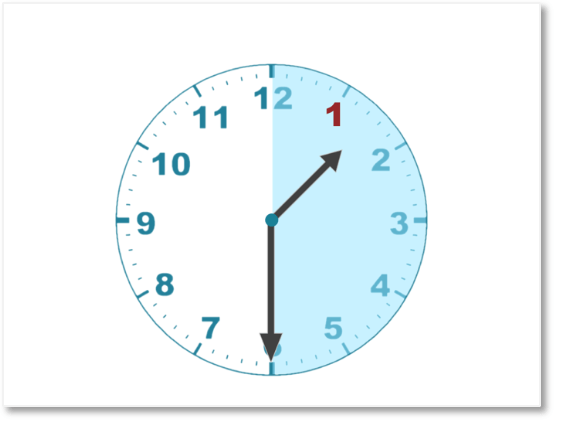error: Content is protected !!